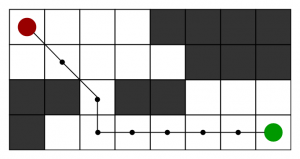• Pinterest

u can watch on : https://drive.google.com/open?id=1zhvaizcb7nnu1ER-TDhEFwUbbVlQtDWB

What is A* Search Algorithm?
A* Search algorithm is one of the best and popular technique used in path-finding and graph traversals.

What A* Search Algorithm does is that at each step it picks the node according to a value-‘f’ which is a parameter equal to the sum of two other parameters – ‘g’ and ‘h’. At each step it picks the node/cell having the lowest ‘f’, and process that node/cell.

We define ‘g’ and ‘h’ as simply as possible below

g = the movement cost to move from the starting point to a given square on the grid, following the path generated to get there.
h = the estimated movement cost to move from that given square on the grid to the final destination. This is often referred to as the heuristic, which is nothing but a kind of smart guess. We really don’t know the actual distance until we find the path, because all sorts of things can be in the way (walls, water, etc.). There can be many ways to calculate this ‘h’ which are discussed in the later sections.

Algorithm

1. Initialize the open list

2. Initialize the closed list put the starting node on the open list (you can leave its f at zero)
3. while the open list is not empty

a) find the node with the least f on the open list, call it “q”

b) pop q off the open list

c) generate q’s 8 successors and set their parents to q

d) for each successor

i) if successor is the goal, stop search successor.

g = q.g + distance between successor and q successor.

h = distance from goal to successor (This can be done using many ways, we will discuss three heuristics- Manhattan, Diagonal and Euclidean Heuristics) successor.

f = successor.g + successor.h

ii) if a node with the same position as successor is in the OPEN list which has a lower f than successor, skip this successor

iii) if a node with the same position as successor is in the CLOSED list which has a lower f than successor, skip this successor otherwise, add the node to the open list end (for loop) e) push q on the closed list end (while loop)